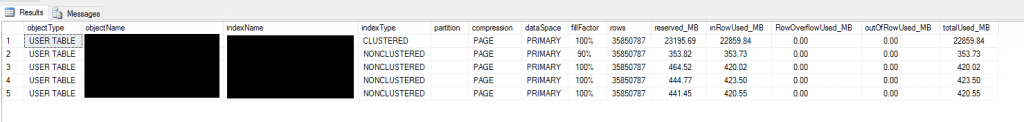# Azure SQL Database Index Size

Recently I’ve been doing some work on optimising indexes within Azure SQL Database. The following SQL from Daniel is very useful in showing the status of all the current indexes within a SQL Database. The SQL works for both SQL Server 2014 onwards, plus Azure SQL Database. I have not tested it on any other versions.

This code returns the following:

• Object Type
• Object Name
• Index Name
• Index Type
• Partition (if any)
• Compression
• Data Space
• Fill Factor
• Rows
• Reserved MB
• In Row Used MB
• Row Overflow Used MB
• Out of Row Used MB
• Total Used MB
```SELECT --- Schema, type and name of object and index:
REPLACE(obj.type_desc, '_', ' ') AS objectType
,       sch.[name] + '.' + obj.[name] AS objectName
,       ISNULL(ix.[name], '') AS indexName
,       ix.type_desc AS indexType
,       --- Partition number, if there are partitions:
(CASE COUNT(*) OVER (
PARTITION BY ps.[object_id]
,ps.index_id
)
WHEN 1
THEN ''
ELSE CAST(ps.partition_number AS VARCHAR(10))
END) AS [partition]
,       --- Storage properties:
p.data_compression_desc AS [compression]
,       ds.[name] + ISNULL('(' + pc.[name] + ')', '') AS dataSpace
,       STR(ISNULL(NULLIF(ix.fill_factor, 0), 100), 4, 0) + '%' AS [fillFactor]
,       --- The raw numbers:
ps.row_count AS [rows]
,       STR(1.0 * ps.reserved_page_count * 8 / 1024, 12, 2) AS reserved_MB
,       STR(1.0 * ps.in_row_used_page_count * 8 / 1024, 12, 2) AS inRowUsed_MB
,       STR(1.0 * ps.row_overflow_used_page_count * 8 / 1024, 12, 2) AS RowOverflowUsed_MB
,       STR(1.0 * ps.lob_used_page_count * 8 / 1024, 12, 2) AS outOfRowUsed_MB
,       STR(1.0 * ps.used_page_count * 8 / 1024, 12, 2) AS totalUsed_MB
FROM sys.dm_db_partition_stats AS ps
INNER JOIN sys.partitions AS p ON     ps.[partition_id] = p.[partition_id]
INNER JOIN sys.objects AS obj ON     ps.[object_id] = obj.[object_id]
INNER JOIN sys.schemas AS sch ON     obj.[schema_id] = sch.[schema_id]
LEFT JOIN sys.indexes AS ix ON     ps.[object_id] = ix.[object_id]
AND     ps.index_id = ix.index_id
--- Data space is either a file group or a partition function:
LEFT JOIN sys.data_spaces AS ds ON     ix.data_space_id = ds.data_space_id
--- This is the partitioning column:
LEFT JOIN sys.index_columns AS ixc ON     ix.[object_id] = ixc.[object_id]
AND     ix.index_id = ixc.index_id
AND     ixc.partition_ordinal > 0
LEFT JOIN sys.columns AS pc ON     pc.[object_id] = obj.[object_id]
AND     pc.column_id = ixc.column_id
--- Not interested in system tables and internal tables:
WHERE obj.[type] NOT IN (
'S'
,'IT'
)
ORDER BY sch.[name]
,obj.[name]
,ix.index_id
,p.partition_number;
```

Example Results:This site uses Akismet to reduce spam. Learn how your comment data is processed.